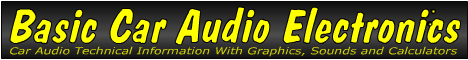Email   Home Page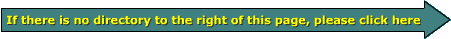x

 Web www.bcae1.com www.bcot1.com
Use F11 to go to full screen
Translate.

Bridgeable Amplifiers

Amplifier bridging is simply using 2 channels of an amplifier to drive a common load. For 2 channel amplifiers, one left signal and one right signal is used to drive a mono speaker load. Keep in mind that mono and bridging are not necessarily the same. Mono means that there's only one output signal. There could be more than one speaker but each speaker will have the same output. Bridging means that you are using more than one source of power to drive a load (speaker). The sources of power are the independent channels of the amplifier.

To bridge amplifiers that have signal only on the positive speaker terminals, if you want to bridge two channels to one load, you have to use some means to invert the signal on one channel (remember the old 'bridging modules' for Orion amplifiers?). Today's bridgeable multi-channel amplifiers (2 or more independent channels) have an inverted channel or an on-board switch (to invert the output of the amp) as part of their design to allow bridging. For many amplifiers, the left positive and right negative are are the signal outputs. A few use the left negative and the right positive. Mono amplifiers that have only one channel must have a way to invert the signal on the output of the amplifier. To bridge two mono amps, you invert the output of one of the two amplifiers and connect one amplifier's output to the positive speaker terminal and the other amplifier to the other speaker terminal.

Note:
Before we go any farther let me say this... It it NOT necessary to bridge a multi-channel amplifier to make it produce maximum power. Bridging is simply one option. If an amplifier is 2 ohm stereo stable (and therefore 4 ohm mono stable), it will produce the same power into a 2 ohm stereo load as it will into a 4 ohm mono load.

Many people feel that they have to connect every multi-channel amplifier they own (generally 2 ohm stereo stable or 4 ohm mono stable) in a 2 ohm mono configuration. These are generally the same people who have owned (and destroyed) many amplifiers. This is because very few amplifiers (especially Class A/B amplifier) are capable of safely driving a 2 ohm mono load. The reason why a 2 ohm mono configuration is dangerous to most multi-channel amplifiers will be covered a bit later.

As was stated previously, bridging an amplifier simply means using two output terminals both of which have signal on them (there's usually one each from the left and right channels of the amplifier). It is usually done to increase the power output to a speaker or to utilize both channels of a stereo amplifier if you only have one woofer.

To fully understand how amplifiers are bridged, we should first cover "phase" as it applies to audio amplifiers. The following diagram shows 3 sine waves that are out of phase with each other, to varying degrees.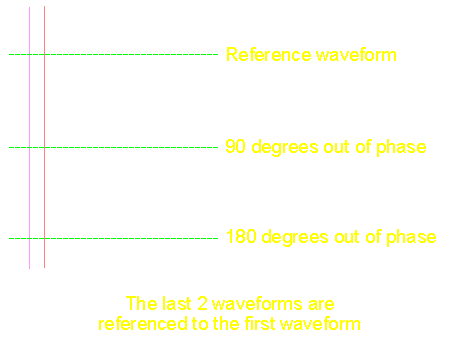As you can see, the first waveform is the reference. You must have a reference or the term "phase" has no meaning. In the above diagram, the middle waveform is 90 degrees out of phase with respect to both of the other waveforms. The third waveform is 180 degrees out of phase with respect to the reference waveform and 90 degrees out of phase with respect to the middle waveform. The diagram below shows the phase angles in a different type of illustration.

The following diagram shows how the waveform relates to the 360 degrees of a complete circle (one complete cycle of the waveform).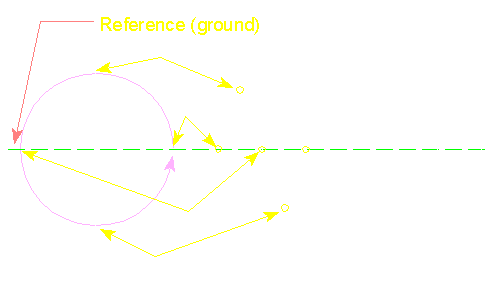In diagram B:

• The waveform's potential (voltage) is at (equal to) ground (the reference) which, in this case, is the same as "0 degrees". The instantaneous voltage increases as the waveform moves toward 90 degrees.
• At this point, the waveform has gone through 90 of the 360 degree cycle. This is the point of maximum instantaneous voltage for the sine wave signal. After it passes this point in a counter clockwise direction, the voltage starts to drop. Note that at this point, the speaker would be forced the maximum distance from its point of rest. For most speakers, if this signal were applied to the positive terminal of the speaker and the negative terminal were connected to the reference (ground), the speaker would be pushed out of the box.
• At point c, the instantaneous voltage is back at reference and we have gone through 180 of the 360 degree total cycle. If another sine wave of the same frequency would start at "A" at this point in time, it would be 180 degrees out of phase with reference to the original waveform. The speaker (mentioned above) would be back at its point of rest at this point in time.
• This is 270 degrees through the cycle. You can see that the voltage is at it's lowest point (it's actually at its maximum negative instantaneous voltage). At this point in time, the speaker would be pulled in. The voltage will start to increase as it moves through this point. When the waveform reaches point "A", it starts a new cycle.

This diagram shows 2 waveforms and the reference to the 360� cycle.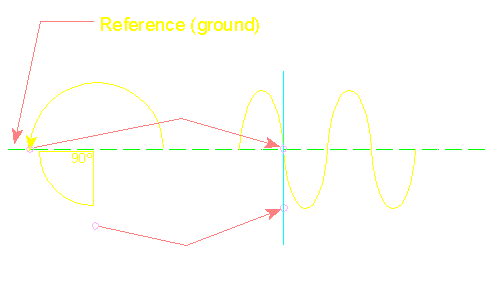And this diagram shows two sine waves that are 180� out of phase.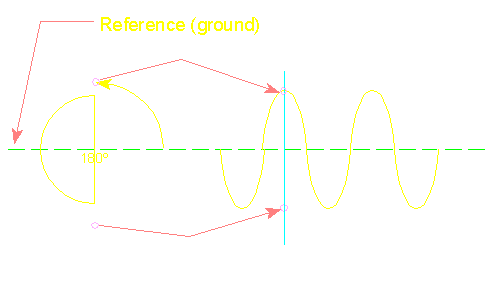In the diagram below, there are 2 sine waves. The first waveform is the "normal" sine wave. The bottom waveform is "inverted" or 180 degrees out of phase with respect to the "normal" signal. In a 2 channel (left and right) bridgeable amplifier, one output is in phase with the input signal and the other channel is inverted. In most bridgeable amplifiers, especially American made amps, the left positive has the in phase signal and the right negative has inverted signal.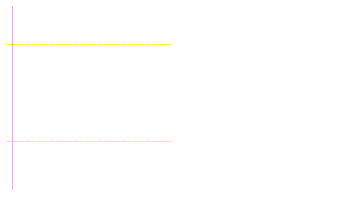In the next image, you can see that both negative terminals, on the non-bridgeable amp, go to a reference point inside of the amp. This means that there is no signal on those connections. In many amplifiers, the reference is actually 'ground'. You will also see that both positive terminals have a normal (non-inverted) signal on them.

In contrast...
The left channel of the bridgeable amplifier is set up just like the left channel of the non-bridgeable but the right channel of the bridgeable amp is where you will see the difference. Notice that the right negative has the signal on it, also notice that the signal is inverted (flipped upside down).

In the following diagram, you can see a speaker connected in a normal configuration and another speaker connected in bridged mode. You can see that the peak voltage available to the normal speaker is 1/2 the voltage available to the bridged speaker (between points A and B). The normal connection uses 1 signal lead and the reference (ground). The bridged speaker uses 2 signal leads. Since the voltage available to the bridged speaker is doubled (between points C and D), the power driven into the speaker can be four times as much as the normal connection (remember that P=E2/R).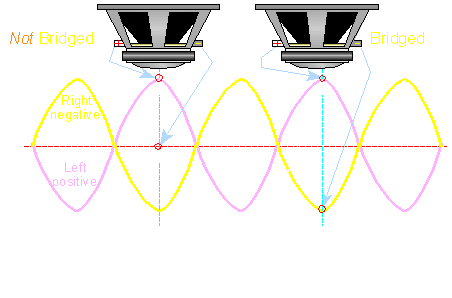Refresher:
Remember that the AC voltage across a speaker's voice coil is what determines the amount of power dissipated by the voice coil (and ultimately how much sound pressure the speaker will produce). In other words, when more voltage is applied to a speaker, the speaker will play louder. If one speaker terminal is connected to a reference point which has no signal (commonly referred to as ground-indicated by the red line) and the other speaker terminal is connected to the signal (speaker output) lead of the amplifier, you will only be able to get half of the power supply's total voltage across the speaker at any point in time.

Realize that a speaker must move equally in both directions from its point of rest. If the amplifier's power supply output is 40 volts total or �20 volts with "ground" as the reference, the maximum instantaneous voltage that can be applied across the non-bridged speaker's terminals is 20 volts. Well this would be true if the amplifier components were 100% efficient. In the real world the output voltage would be somewhat lower due to inefficiencies but we won't worry about inefficiency right now. For now assume that the full power supply voltage (positive or negative) can be driven into the speaker. If you remember the section on Ohm's law, you will understand that the amp will only be able to produce 100 watts (peak power) into a 4 ohm speaker.

P=E*E/R
Power=(voltage across speaker*voltage across speaker)/(resistance of the speaker)
Power=(20*20)/4 ohms
Power=100 watts

NOTE:

1. I used resistance instead of impedance because, for this example, impedance would complicate things greatly.
2. Even with 100% amplifier efficiency, The RMS power would only be 1/2 of the power output indicated. The 100 watts is peak power.

Now, what if you have only a single 4 ohm speaker and a 2 channel NON-bridgeable amplifier with sufficient current output capability to drive a 2 ohm load on each of its output channels? You know that the amplifier could produce MUCH more (and also maximum) power into four 4 ohm speakers (which would be equal to a 2 ohm load per channel), but maximum power would not be produced into the single 4 ohm speaker on a single output channel (i.e. left OR right). To produce maximum power into a single 4 ohm speaker (without increasing the rail voltage) you could simply invert the signal of one channel and bridge the speaker on the amplifier. This is why some of the older amplifiers used a 'bridging module' (it inverted the signal going to one channel). It is very easy to invert one channel when designing an amplifier and it makes the amp much more versatile. When one channel is inverted, it's output voltage is of the same magnitude as the "normal" channel but is of opposite polarity (as indicated by the violet and yellow lines of the following diagram).At any point in time, if the normal channel's output voltage is positive, the inverted channel's output voltage is negative and vice-versa. The inverted channel is basically a mirror image of the normal channel. Now remember that 4 ohm speaker and the fact that the power dissipated in it's voice coil is determined by the voltage across its terminals. With the bridgeable amp, one of the speaker's terminals would be connected to the normal output channel (violet waveform) and the other speaker terminal would be connected to the inverted channel (yellow waveform) of the amplifier. This allows you to get the total power supply voltage across the speaker. Remember, we are not concerning ourselves with inefficiencies within the amplifier. You can see by the following formula, that the power getting to the speaker is much greater.

P=E*E/R
Power=(40*40)/4 ohms
Power=400 watts

You can see that this is considerably more power! (100 watts un-bridged and 400 watts bridged)

VERY IMPORTANT...

If a bridgeable amplifier is rated to drive a 4 ohm minimum load on each of its channels, it WILL fail if you try to drive a 4 ohm bridged mono load. If you have an amplifier rated to drive a 2 ohm stereo load (2 ohms on each channel), it's only going to be able to drive a 4 ohm (or higher) bridged mono load. A 2 ohm bridged mono load will more than likely destroy the amplifier. Too many people think that all large amplifiers can drive low impedance loads. In general, only amplifiers designated high-current and class D amps can drive low impedance loads. Even class D amplifiers have a limit as to the lowest impedance and when bridged with a second amplifier, the rated impedance is 2x the rated impedance when run normally (not bridged with a second amplifier).

2 Ohm Stereo vs 4 Ohm Mono Loads

There seems to be some confusion as to why a 4 ohm mono and a 2 ohm stereo load are the same, as far as the amplifier is concerned. When two 4 ohm speakers are connected to each channel of a 2 channel amplifier, the amplifier is capable of driving the speakers with half of the total power supply voltage. If the amplifier has a power supply which produces plus or minus 20 volts, it will not be able to drive the speakers on a single channel with any more than 20 volts at any point in time. If we have a 2 ohm load on each channel, at the highest point on the waveform the amplifier will apply 20 volts to the speaker load. Remember that we are only considering a single point in time for this example. If we go back to ohms law...

I=V/R
I=20/2
I=10 amperes

If we take a single 4 ohm speaker and bridge it on that same amplifier, the amplifier will be able to apply twice the voltage across the speaker. This is because while one speaker terminal is being driven positive (towards the positive rail), the other terminal is being driven towards the negative rail. This will allow the entire power supply voltage to be applied to the speaker's voice coil. It will now be able to drive the 4 ohm speaker with 40 volts instead of 20 volts in the previous example. Back to Ohm's law...

I=V/R
I=40/4
I=10 amperes

The same amount of current flows through the output transistors whether the amplifier is driving a 4 ohm mono load or 2 ohm stereo load. As far as the amplifier is concerned, they are the same load.

NOTE:
Some people say that when an amplifier is bridged onto a 4 ohm load, it 'sees' a 2 ohm load. While it is true that the same current flows whether the amp is bridged on a 4 ohm load or a 2 ohm stereo load, the amplifier is driving a 4 ohm load across its outputs. A single 4 ohm speaker can never be a 2 ohm load.

As was previously stated, a 4 ohm mono load is the same as a 2 ohm stereo load as far as the amplifier is concerned. Looking at the next diagram, configuration 'x' shows a 2 ohm stereo load. Configuration 'y' shows a 4 ohm mono load. If both amplifiers are driven to the same output level, the current flowing through the outputs would be equal. In configuration z we have added another 4 ohm speaker bridged onto the amplifier. This means that the load has doubled which means that there is only one half of the impedance of configuration 'y'.

Remember that resistance/impedance is the opposition to the flow of electrical current. An amplifier depends on a high enough impedance to limit the current flowing through the output transistors when the amplifier drives it's full rail voltage into the load. When the load is lowered to 2 ohms mono as in configuration z, the current flow through the output transistors is doubled (4 ohms mono vs 2 ohms mono). The amplifier's maximum safe output current may be only slightly higher than that needed to drive a 4 ohm mono or 2 ohm stereo load. When the amplifier tries to drive the 2 ohm mono at full rail voltage (40 volts) the safe operating area of the transistors will (more than likely) be exceeded. Keep in mind that we are talking about the most common types of amplifiers which are designed to drive 2 ohms or higher per channel (2 ohm stereo stable and 4 ohm mono stable amplifiers). There are amplifiers (high current amplifiers) which are designed to drive low impedance loads. These amplifiers have more transistors and heavier duty components to withstand the increased flow of current through the output transistors.

 You should remember: When a speaker is bridged onto an amplifier, BOTH speaker terminals are driven with a signal. The signal on one speaker terminal is a 'normal' signal while the signal on the other speaker terminal is 'inverted'

```

```